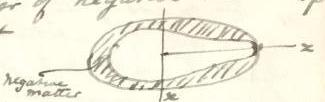## 3-15-23. George Howard Darwin to H. Poincaré

Aug. 12. 01

Newnham Grange–Cambridge

Dear Monsieur Poincaré,

I enclose your former letter.11endnote: 1 Poincaré had asked Darwin to return his letter (§ 3-15-19), of which he had no copy; see Poincaré to Darwin (§ 3-15-22). I do not understand your “double couche”.

With regard to the integrals they are obviously all reducible to elliptic integrals but the coeffts become complicated. In applying them it is necessary to separate the variables $\theta$ and $\varphi$ & this leads to a terrible complication. I have worked for a long time at the mass of the layer to cubes of small quantities. I found that I could not get it simpler than in a form involving 44 integrals occurring in pairs each of which will involve $E$, $F$, $\Pi$. I dare say I am very clumsy at this work but I have given up that attempt & am now arranging the work quite otherwise but less accurate. It will remain very heavy but within the limits of patience.

I am still confident of my correctness & my confidence is largely due to the fact that the problem I have solved is done correctly & the analogy is perfect.

This is my procedure.

Let $\omega^{2}$ be sq. of ang. vel. of the critical Jacobian and $\omega^{2}+\varpi^{2}$ that of the pear. Define the pear as an ellipsoid, touching the stalk of the pear, similar to the critical Jacobian, with a layer of negative matter $-\rho$ lying on it.Let $\omega^{2}$ be sq. of ang. vel. of the critical Jacobian and $\omega^{2}+\varpi^{2}$ that of the pear. Define the pear as an ellipsoid, touching the stalk of the pear, similar to the critical Jacobian, with a layer of negative matter $-\rho$ lying on it.

Let $d$ be distance of center of inertia of pear from origin.

Let $\int_{e}dv$, $\int_{\ell}dv$, denote integration thro’ ellipsoid & thro’ layer and $\int_{e-\ell}dv$ integration thro’ the pear.

The “lost” energy is

 $E=\frac{1}{2}\int_{e-\ell}V_{e-\ell}\rho dv+\frac{1}{2}(\omega^{2}+\varpi^{2})% \int_{e-\ell}[y^{2}+(z-d)^{2}]dv$

Now22endnote: 2 We insert $\rho$ on the left-hand side and in the final term on the right-hand side of the first equation.

 $\displaystyle\frac{1}{2}\int_{e-\ell}V_{e-\ell}\rho dv$ $\displaystyle=\frac{1}{2}\int_{e}V_{e}\rho dv-\int_{\ell}V_{\ell}\rho dv+\frac% {1}{2}\int_{\ell}V_{\ell}\rho dv$ $\displaystyle\int_{e-\ell}\rho[y^{2}+(z-d)^{2}]dv$ $\displaystyle=\int_{e}\rho(y^{2}+z^{2})dv-\int_{\ell}\rho(y^{2}+z^{2})dv-\int_% {e-\ell}(2zd-d^{2})\rho dv$

But

 $\int_{e-\ell}z\rho dv=Md,\qquad\int_{e-\ell}\rho dv=M.$

Therefore

 $\int_{e-\ell}(2zd-d^{2})\rho dv=Md^{2}$

Hence

 $\displaystyle E=\frac{1}{2}$ $\displaystyle\int_{e}V_{e}\rho dv+\frac{1}{2}(\omega^{2})\int_{e}(y^{2}+z^{2})% \rho dv$ $\displaystyle-$ $\displaystyle\int_{l}\left[V_{e}+\frac{1}{2}\omega^{2}(y^{2}+z^{2})\right]\rho dv% +\frac{1}{2}\int_{\ell}V_{\ell}\rho dv$ $\displaystyle+$ $\displaystyle\frac{1}{2}\varpi^{2}\int_{e-\ell}(y^{2}+z^{2})\rho dv-\frac{1}{2% }Md^{2}(\omega^{2}+\varpi^{2})$

$d$ is clearly at least of second order & $d^{2}$ is of fourth order & negligible.33endnote: 3 Variante: “…fourth order & negligible. The first two terms in $E$ are stationary because the ellipsoid is a Jacobian”.

Suppose that the volume of the pear is equal to $\frac{4}{3}\pi\rho k_{0}^{3}abc$, where

 $a=\frac{\cos y}{\kappa\sin y},\qquad b=\frac{\sqrt{1-\kappa^{2}\sin^{2}y}}{% \kappa\sin y},\qquad c=\frac{1}{\kappa\sin y}$

Then $y$ and $\kappa$ have definite numerical values depending on the eccentricities of the principal sections of the critical Jacobian Ellipsoid, so that $a$, $b$, $c$ have known numerical values.

Then it is easy to prove that

 $\displaystyle\frac{1}{2}\int_{e}\rho V_{e}dv+\frac{1}{2}\omega^{2}\int_{e}\rho% (y^{2}+z^{2})dv\\ \displaystyle=\frac{8}{15}\pi^{2}\rho^{2}a^{3}b^{3}c^{3}\cdot k^{5}\left[\int_% {0}^{\infty}\frac{du}{\sqrt{u+a^{2}\cdot u+b^{2}\cdot u+c^{2}}}+\frac{b^{2}+c^% {2}}{abc}\cdot\frac{\omega^{2}}{4\pi\rho}\right]$

In this expression $k$ is such that $kc$ is equal to the distance from the origin at centre of the ellipsoid to the end of the stalk of the pear, and it is therefore a function of the unknown parameter or parameters which define the pear. The only variable in this expression is $k$, all the other quantities including $\omega$ having definite numerical values.

I do not assert that the critical Jacobian is the ellipsoid which most nearly resembles the pear, but I assert that any additional harmonic terms of the second degree must have coefficients of the second order in reference to the parameter which defines the pear.

The pear will also require harmonics of the 1st and 4th degrees to be fully represented, but these harmonics have coefficients of at least the second order, and in the energy only of the fourth order.

We have (as in the case of the Maclaurin ellipsoid the general principle that in developing the energy we need only regard terms of the first order, but the coefficients of those terms must be developed as far as cubes of small quantities in the expression for the energy.

The whole energy as far as material is then:

$\displaystyle{E=\frac{8}{15}\pi^{2}\rho^{2}a^{3}b^{3}c^{3}.k^{5}\left[\int_{0}% ^{\infty}\frac{du}{\sqrt{u+a^{2}.u+b^{2}.u+c^{2}}}+\frac{b^{2}+c^{2}}{abc}% \cdot\frac{\omega^{2}}{4\pi\rho}\right]}$
(Energy of ellipsoid with rotation $\omega$ within itself) $\displaystyle{-\int_{l}\left(V_{e}+\frac{1}{2}\omega^{2}(y^{2}+z^{2})\right)% \rho dv}$ (energy of ellipsoid with rotation $\omega$ and layer) $\displaystyle{+\frac{1}{2}\int_{l}V_{l}\rho dv}$ (energy of layer within itself) $\displaystyle{+\frac{1}{2}\overline{\omega}^{2}\int_{e-l}\rho(y^{2}+z^{2})dv}$ (additional kinetic energy of pear due to change of rotation)

All these quantities are expressible in terms of $e$ and of $k$, and by expressing $k$ in terms of $k_{0}$ we get the whole of $E$ expressed [in] terms of $e$.

Only terms of first order are retained but those terms are developed as far as $e^{3}$.

By differentiating with respect to $e$ we shall get $\varpi^{2}$ in terms of $e$.

In the case of Maclaurin’s ellipsoid of which I have given the solution, $\omega$ is zero so that the terms become:

• 1

Energy of sphere with itself

• 2

Energy of sphere with layer

• 3

Energy of layer with itself

• 4

Kinetic energy of ellipsoid.

Pray excuse the untidiness of this letter.

It will interest me much to hear what your opinion is.

Yours very Sincerely.

G. H. Darwin

ALS 6p. Collection particulière, Paris 75017.

Time-stamp: " 4.05.2019 00:12"

### Notes

• 1 Poincaré had asked Darwin to return his letter (§ 3-15-19), of which he had no copy; see Poincaré to Darwin (§ 3-15-22).
• 2 We insert $\rho$ on the left-hand side and in the final term on the right-hand side of the first equation.
• 3 Variante: “…fourth order & negligible. The first two terms in $E$ are stationary because the ellipsoid is a Jacobian”.